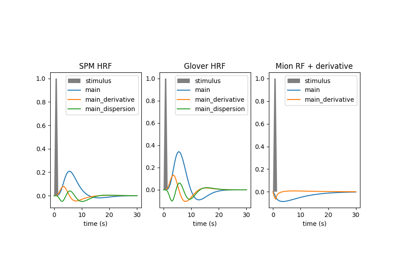Note

This page is a reference documentation. It only explains the function signature, and not how to use it. Please refer to the user guide for the big picture.

# nilearn.glm.first_level.compute_regressor#

nilearn.glm.first_level.compute_regressor(exp_condition, hrf_model, frame_times, con_id='cond', oversampling=50, fir_delays=None, min_onset=-24)[source]#

This is the main function to convolve regressors with hrf model

Parameters:
exp_conditionarray-like of shape (3, n_events)

yields description of events for this condition as a (onsets, durations, amplitudes) triplet

hrf_model`str`, function, list of functions, or None

This parameter defines the HRF model to be used. It can be a string if you are passing the name of a model implemented in Nilearn. Valid names are:

It can also be a custom model. In this case, a function should be provided for each regressor. Each function should behave as the other models implemented within Nilearn. That is, it should take both t_r and oversampling as inputs and return a sample numpy array of appropriate shape.

Note

It is expected that spm standard and glover models would not yield large differences in most cases.

Note

In case of glover and spm models, the derived regressors are orthogonalized wrt the main one.

frame_timesarray of shape (n_scans)

the desired sampling times

con_idstring, optional, default is ‘cond’.

Identifier of the condition

oversamplingint, optional

Oversampling factor to perform the convolution. Default=50.

fir_delays[int] 1D-array-like, optional

Delays (in scans) used in case of a finite impulse response model.

min_onsetfloat, optional

Minimal onset relative to frame_times (in seconds) events that start before frame_times + min_onset are not considered. Default=-24.

Returns:
computed_regressorsarray of shape(n_scans, n_reg)

Computed regressors sampled at frame times.

reg_nameslist of strings

Corresponding regressor names.

## Examples using `nilearn.glm.first_level.compute_regressor`#Example of MRI response functions

Example of MRI response functions